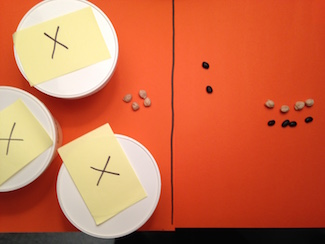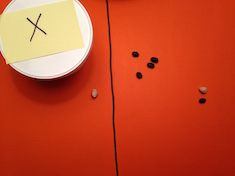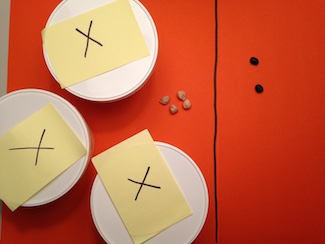By Kathy Kuhl

Today we’ll finish looking at bean algebra, a simple way to make algebra concrete. We’ll start using negative numbers with our bean algebra. (This 4-part series began here.)

Often we rush our children into abstraction too soon when we teach math. But if our children practice at length with concrete objects, illustrate their discoveries, and write them down in algebra, it will make more sense. “Build it, draw it, write it,” and they are more likely to understand and to remember. (I discuss this in my math workshops; come hear me to learn more.)

When beginning algebra, we’re not going to include any fractions or decimals when we teach these new concepts, just integers. (Remember that integers are the whole numbers and their opposites.)

Quick review of earlier principles:• Teach solving equations like puzzles. Search for the mystery number. The person inventing the puzzle (equation) begins by choosing a solution and putting that many beans in each container, snapping the lids on before showing the puzzle to others to solve. Let your kids build problems and see if they can stump you.
• White beans represent positive integers. Black beans represent negative integers. (If you use poker chips, use red for negative, since “in the red” means negative.)
• Both sides of the problem have the same value. We draw a line on a large piece of paper, put a ruler down the middle of the paper, or use two box lids, one for each side of the equation.
• Keep the two sides equal. When you change the value on one side, like adding 3 or dividing by 2 or tripling), you must do the same to the other side.
• Anti-matter matters! Remember the analogy (from Harold Jacobs’ Elementary Algebra) that we treat negative numbers like bits of anti-matter. When a bit of matter and antimatter come together, they destroy each other. (Kerboom!) It’s more fun than saying -1 + 1 = 0 or 7 + -7=0. (See this post if you don’t recall it.)

### To begin, we’ll construct a couple simple bean algebra problems…

with solutions that are negative. If you’re going to make up bean algebra problems for your children to solve, and if they’re going to make them up to challenge you and their siblings or classmates, you have to begin by choosing your solution.

For this first problem, I chose x = -10. I’ll leave the lids off the containers while building this so you can see that both sides are equal.

Remember to treat both sides the same. I decided to double both sides. So 2x = -20.2x = -20 (We’ll put lids on before we give the equation to someone to solve.)

Next I’ll add -5 to each sides.

Now I snap on the lids, invite my child in to see the puzzle and see if he or she can solve it. First, the child would remove -5 from each side, leaving:Then the child would divide the -20 beans into two groups and tell me I have -10 beans in each container. That’s very much like the equations in earlier posts with positive integers.

But students sometimes struggle with integer arithmetic when we add or subtract positive and negative numbers. So let’s build an equation with them. This time, I chose x = -5. Watch what happens when I add positive 1 to each side.One positive bean cancels out one negative bean (annihilates, obliterates, destroys—great time to throw in vocabulary here) leaving:How doe someone solve this? Suppose you put the lids on and your child walks in to solve the problem:

The child tries to remove a white bean (positive number) from each side. But there is no white bean to remove from the right side!

However, this child remembers that adding one plus negative one is the same as adding nothing, so she adds a black and white bean to the right side:Before those two bean annihilate each other, she can remove +1 (a white bean) from each side, leaving x = -5.

Finally, I’ll help you solve an equation without showing you the solution first.We want to get those containers on one side by themselves so we can figure out what is in each of them. So we need to take away those 4 positive beans from each side.

But there are none to take on the right. So, we add 4 positive and 4 negative beans to the right. That won’t change the value of the right side, because they cancel each other out.

But before those beans explode, we remove 4 positive beans from each side, leaving

3x = -6

Now we divide the beans on the right into three equal groups, and we see there must be -2 in each container.

Try these and build your own. Let your child who hasn’t mastered simple linear equations build them.

Remember to draw them and write them as shown last time. Be sure to use small circles for positive numbers and small black dots for negative numbers, like this. The last problem would look like this. (I copied the drawing at 4 different stages, so you can see how it would change.)